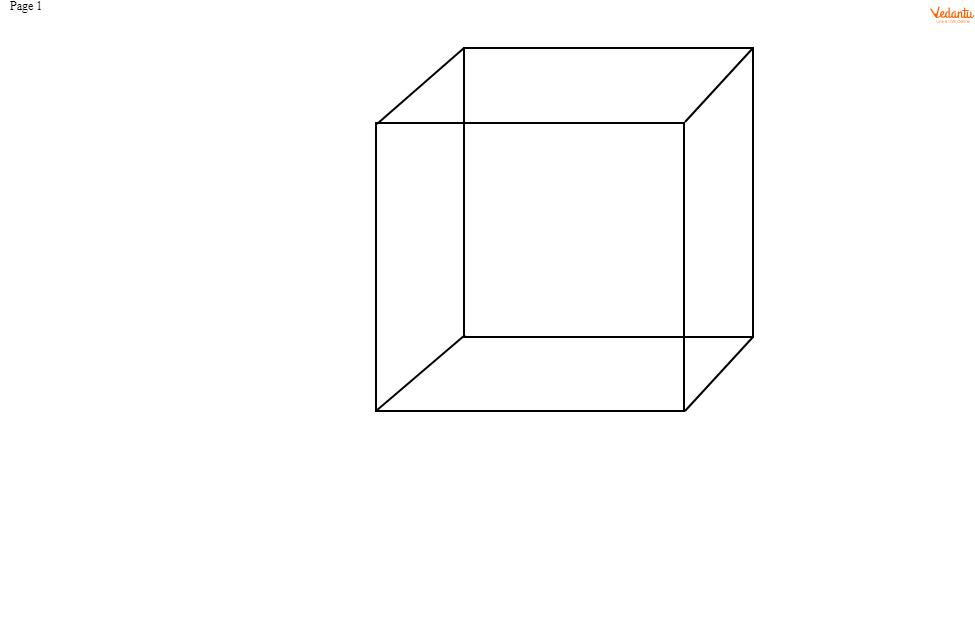QuestionAnswers

# Two cubes have their volumes in the ratio $1:27$ . Find the ratio of their surface areas.

Hint-
In this particular type of question we need to proceed by expressing the sides of the cubes as a and b and finding the ratio between them. Then we need to use it in finding the ratio of surface area of both the cubes.Let the sides of the cube be a and b.
Then,
We know that volume of a cube = ${side^3}$
Therefore ratio of the volumes = $\dfrac{{{a^3}}}{{{b^3}}}$
But ratio = $1:27$
$\Rightarrow \dfrac{{{a^3}}}{{{b^3}}} = \dfrac{1}{{27}} \\ \Rightarrow {\left( {\dfrac{a}{b}} \right)^3} = {\left( {\dfrac{1}{3}} \right)^3} \\ \Rightarrow \dfrac{a}{b} = \dfrac{1}{3} \\$
Therefore,
Ratio of the surface areas = $\dfrac{{6{a^2}}}{{6{b^2}}}$
(since surface area of the cube = $6 \times sid{e^2}$ )
$= \dfrac{{{a^2}}}{{{b^2}}} \\ = {\left( {\dfrac{a}{b}} \right)^2} \\ = {\left( {\dfrac{1}{3}} \right)^2}{\text{ }}\left( {{\text{putting value of }}\dfrac{a}{b}{\text{ from above}}} \right) \\ = \dfrac{1}{9} \\ = 1:9 \\$
﻿﻿﻿Therefore ratio of the surface areas ﻿=$1:9$.

Note- Always recall the formula of volume and surface area of three dimensional figures like cube. Note that a cube is a three-dimensional solid object bounded by six square faces, facets or sides, with three meeting at each vertex.